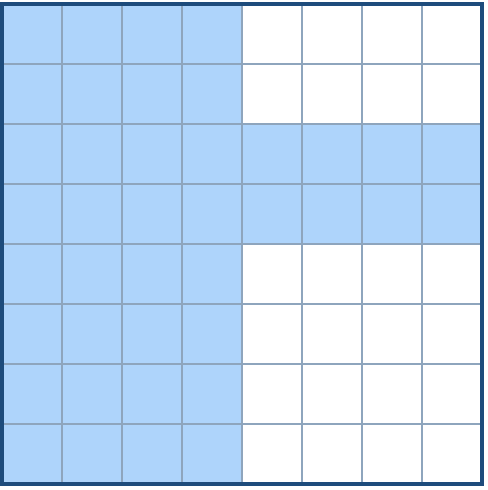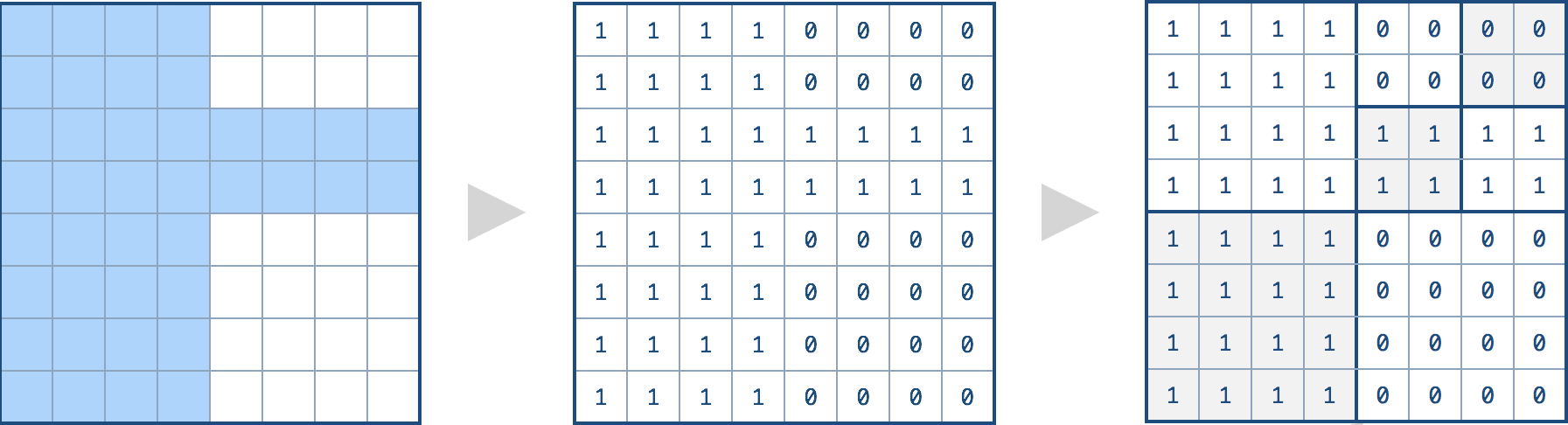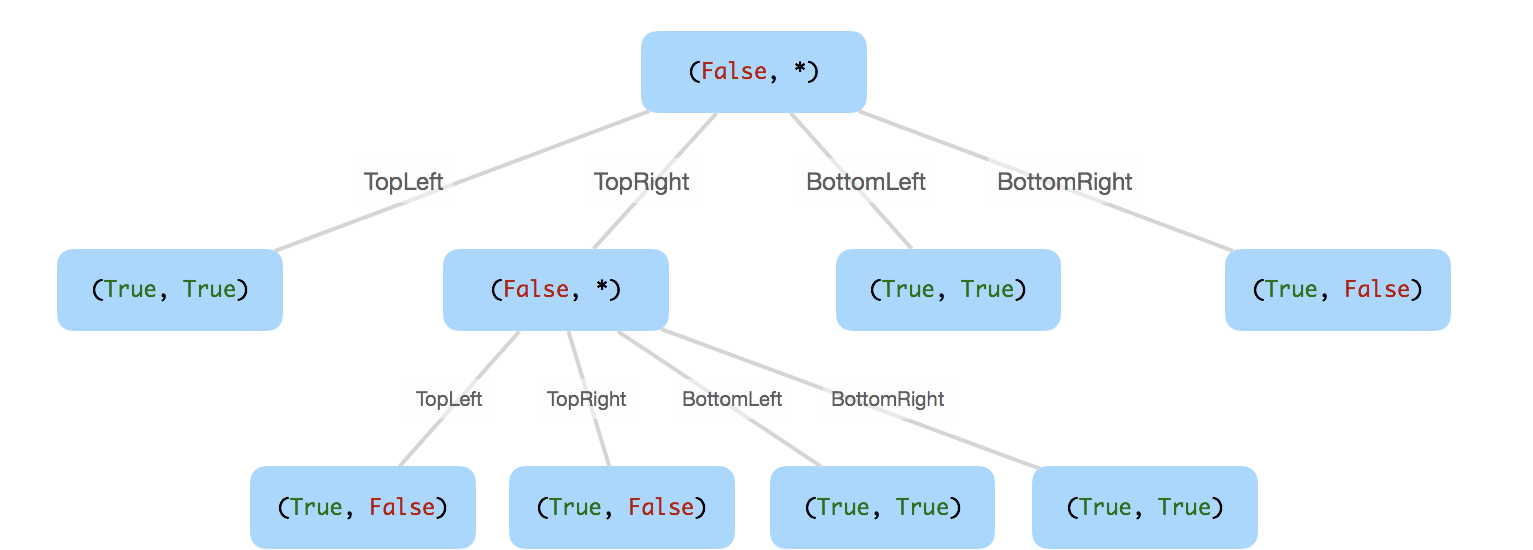quad trees, data structure

We want to use quad trees to store anN x N boolean grid. Each cell in the grid can only be true or false. The root node represents the whole grid. For each node, it will be subdivided into four children nodes until the values in the region it represents are all the same.

Each node has another two boolean attributes :isLeafandval.isLeafis true if and only if the node is a leaf node. Thevalattribute for a leaf node contains the value of the region it represents.

Your task is to use a quad tree to represent a given grid. The following example may help you understand the problem better:

Given the8 x 8grid below, we want to construct the corresponding quad tree:It can be divided according to the definition above:The corresponding quad tree should be as following, where each node is represented as a(isLeaf, val)pair.

For the non-leaf nodes, valcan be arbitrary, so it is represented as*.Note:

1. N is less than 1000 and guaranteened to be a power of 2.
2. If you want to know more about the quad tree, you can refer to its wiki.

Solution

• 判断isLeaf：这里用每个square的左上角作为val，循环该square内所有元素，如果有不同元素，说明还需要继续拆分square，也就是isLeaf = false；否则如果全部元素都是相同的，说明isLeaf = true
• recursively build：在于如果isLeaf = false时，如何对sqaure进行分割，这里可以借鉴binary search的计算，寻找每个square的区间中值，作为细分square的坐标。
/*
// Definition for a QuadTree node.
class Node {
public boolean val;
public boolean isLeaf;
public Node topLeft;
public Node topRight;
public Node bottomLeft;
public Node bottomRight;

public Node() {}

public Node(boolean _val,boolean _isLeaf,Node _topLeft,Node _topRight,Node _bottomLeft,Node _bottomRight) {
val = _val;
isLeaf = _isLeaf;
topLeft = _topLeft;
topRight = _topRight;
bottomLeft = _bottomLeft;
bottomRight = _bottomRight;
}
};
*/
class Solution {
public Node construct(int[][] grid) {
return build(grid, 0, 0, grid.length - 1, grid.length - 1);
}
public Node build(int[][] grid, int r1, int c1, int r2, int c2) {
// optional
if (r1 > r2 || c1 > c2) {
return null;
}

if (isLeafNode(grid, r1, c1, r2, c2)) {
return new Node(grid[r1][c1] == 1, true, null, null, null, null);
}

int rmid = r1 + (r2 - r1) / 2;
int cmid = c1 + (c2 - c1) / 2;

return new Node(false, false,
build(grid, r1, c1, rmid, cmid),
build(grid, r1, cmid + 1, rmid, c2),
build(grid, rmid + 1, c1, r2, cmid),
build(grid, rmid + 1, cmid + 1, r2, c2));
}

private boolean isLeafNode(int[][] grid, int r1, int c1, int r2, int c2) {
int val = grid[r1][c1];

for (int i = r1; i <= r2; i++) {
for (int j = c1; j <= c2; j++) {
if (val != grid[i][j]) {
return false;
}
}
}

return true;
}
}